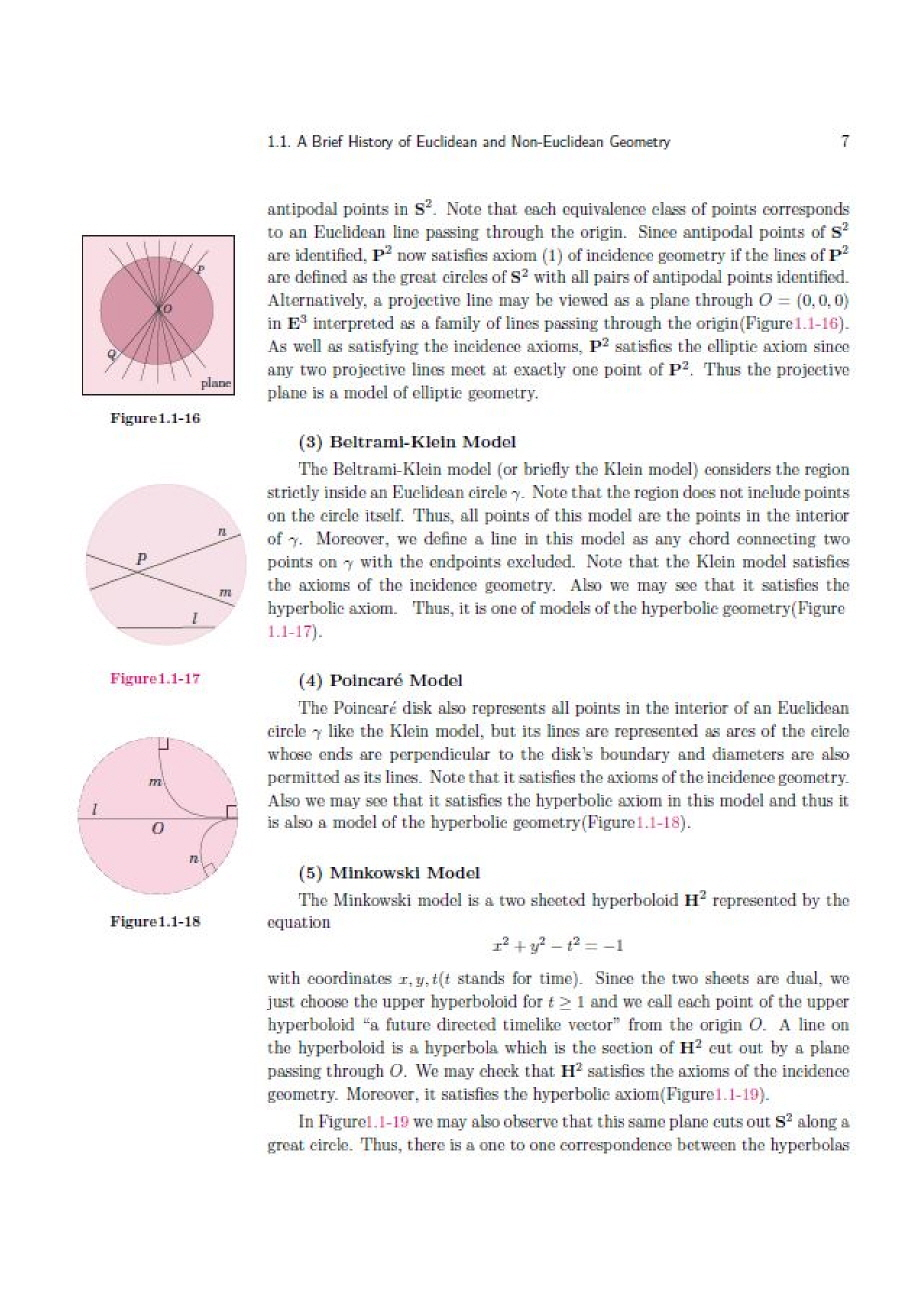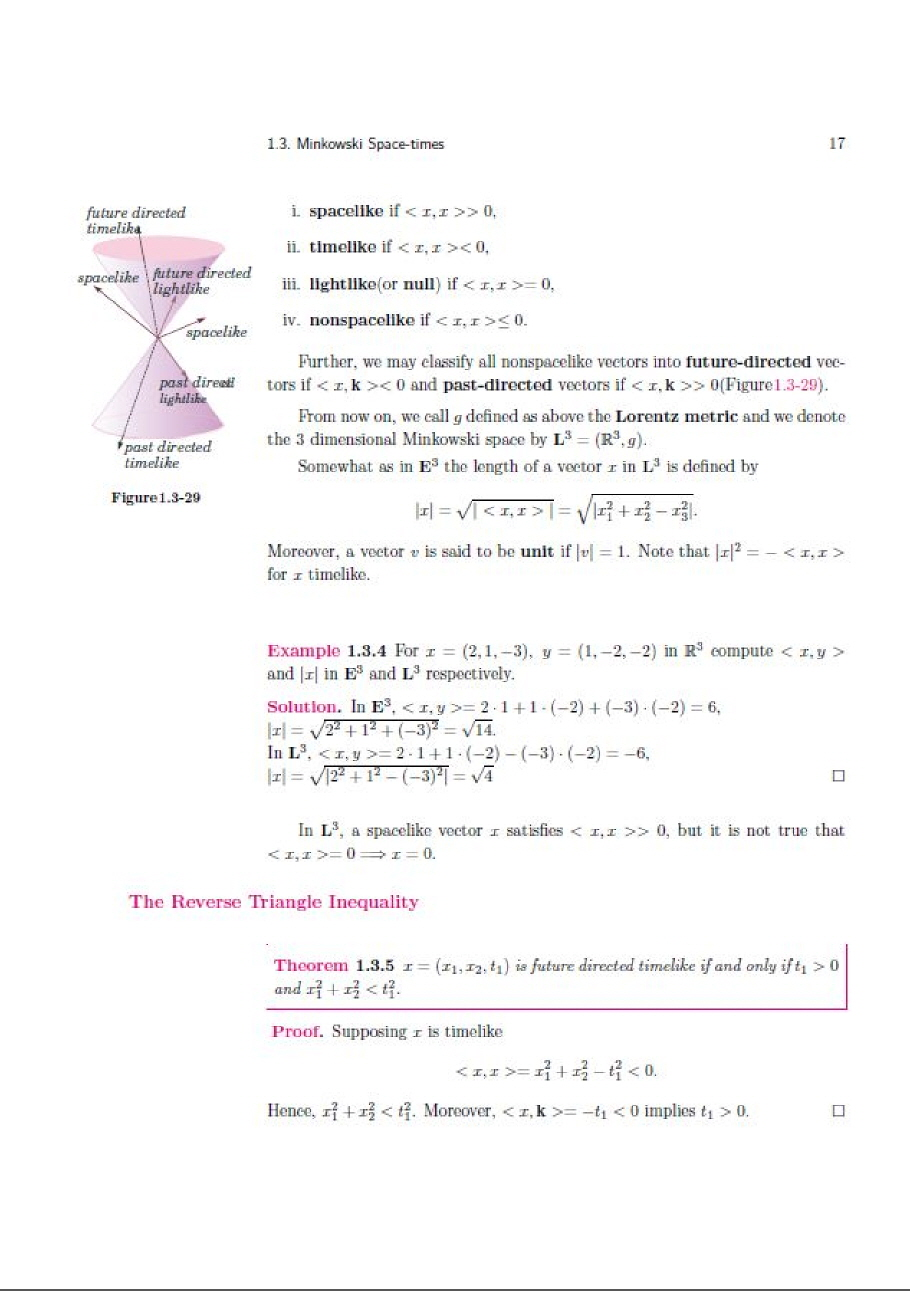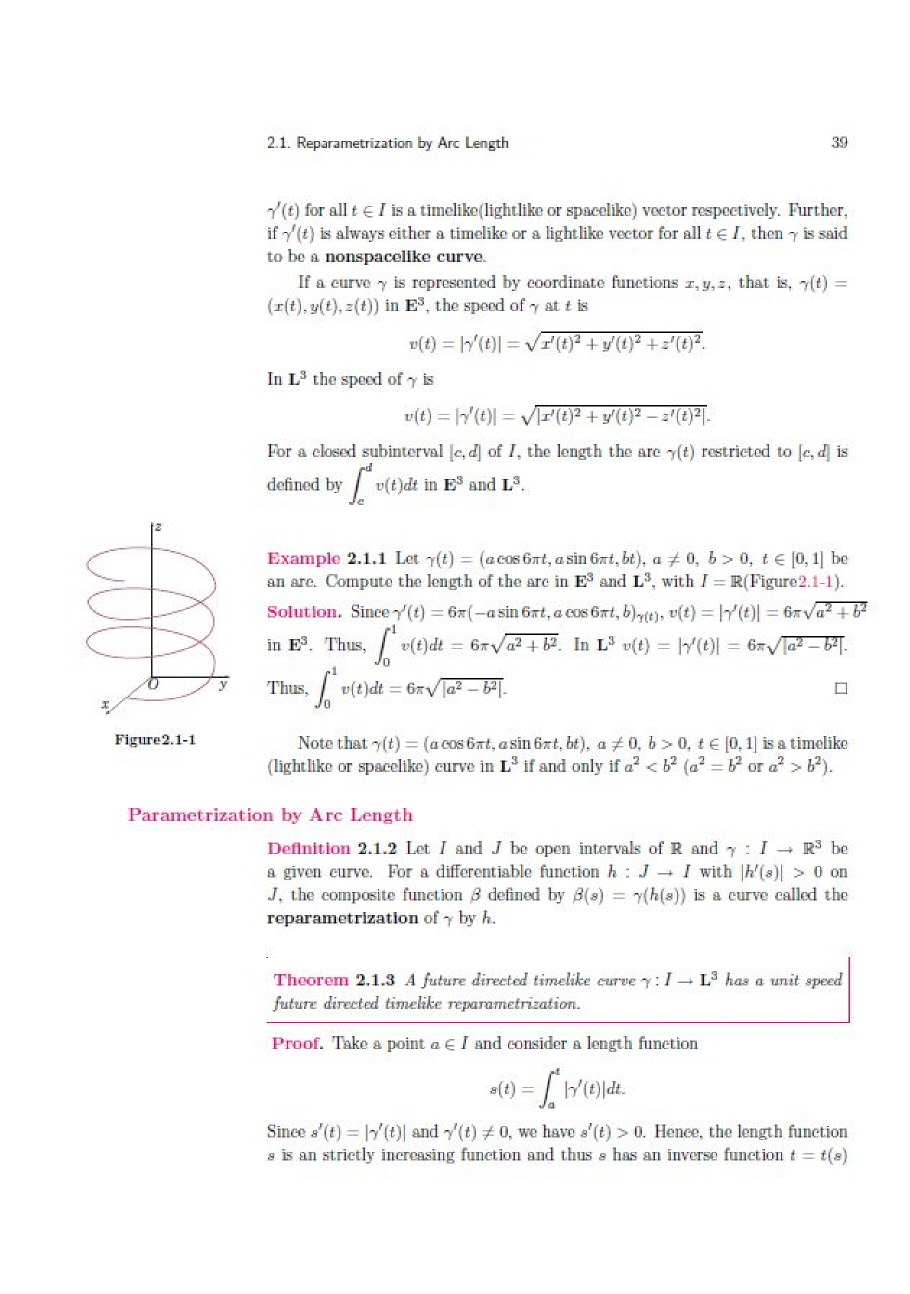## 로그인이필요합니다## 도서를 검색해 주세요.

원하시는 결과가 없으시면 문의 주시거나 다른 검색어를 입력해보세요.

•상품상세

## Differential Geometry in Euclidean and Minkowskian Spaces 요약정보 및 구매

상품 선택옵션 0 개, 추가옵션 0 개

사용후기 0 개
지은이 Paul E. Ehrlich, Seon-Bu Kim 2013-05-01 1판 250 9788961056403 구매가능 18,000원 0점 주문시 결제

### 선택된 옵션

• Differential Geometry in Euclidean and Minkowskian Spaces
+0원

## 관련상품

• This book is about dirential geometry for curves and surfaces in three dimensional Euclidean and Minkowskian spaces.
In chapter 1, we sum up the history of Euclidean and non-Euclidean Geom-etry and introduce the vector lds which are the basic tools to study the theory of curves and surfaces in disrential geometry. In addition, we study the basic ge-ometric properties of Minkowskian space and the hyperbolic trigonometry which plays a similar role in Minkowskian space to the usual trigonometry in Euclidean space.
In chapter 2, we study the Frenet-Serret apparatus for the reparametrization of a regular curve by arc length and introduce the curvature and torsion along the curve. Moreover, we classify curves by their curvature and torsion in three cases, Euclidean curves and both timelike and spacelike curves in Minkowskian space.Further, we need to study the rotation index which will be used as a theorem of turning tangents in the proof of the Gauss-Bonnet Theorem in chapter 5.
In chapter 3, we formulate the concept of regular surface covered by parametrized patches and give examples of surfaces with Maple 칐ures. To measure the cur-vature of a patch for a surface in Euclidean and Minkowskian spaces, we need to compute the 칞st and second fundamental forms of Euclidean surfaces and both timelike and spacelike surfaces.
In chapter 4, we study the shape operator and compute the normal curva-tures, principal curvatures, Gaussian and mean curvatures of a patch for Eu-clidean surfaces and both timelike and spacelike surfaces. Moreover, we observe the invariant properties of curvatures by isometries between two surfaces. Fur-ther, we study the parallel translation and geodesics of the surfaces in Euclidean and Minkowskian spaces.
In chapter 5, we study the Gauss-Bonnet theorem, one of the most important theorems in mathematics, which shows that the geometrical property "Gaussiancurvature" on a compact surface in Euclidean space is related to the topological property "Euler number". But in Minkowskian space we just apply the theory for an interior region of a unit speed timelike or spacelike n-polygonal curve.
Acknowledgements
In 2004, the second author visited the mathematics department of the Uni-versity of Florida for research with the 칞st author to prove volume comparison theorems for Lorentzian manifolds and they decided to write this book for the undergraduate and graduate students. After the second author came back to Chonnam National University in Korea, they continued their writing by using email, with the second author sending the original drafts of the materials for revisions to the 칞st author and then typed his returned corrections by the La-Tex program. The second author received some 칗ancial aid for publishing from Chonnam National University in 2012.
The authors wish especially 칞st to thank the patience of both their families and of the University of Florida and Chonnam National University for many years of  encouragement as this book was developed, and secondly to Kyungmoon Publishers in Seoul in Korea for bringing the book to fruition.

* 2014년도 대한민국학술원 선정 우수학술도서

• 1 Preliminaries . 1
1.1 A Brief History of Euclidean and Non-Euclidean Geometry . 1
1.2 Tangent Vectors and Vector Fields . 9
1.3 Minkowski Space-times . 14
1.4 Hyperbolic Trigonometry . 28

2 Theory of Curves and Vector Calculus . 38
2.1 Reparametrization by Arc Length . 38
2.2 The Curvature and Torsion of Curves . 52
2.3 Vector Calculus . 62
2.4 The Global Theory of Plane Curves . 76

3 Calculus of Surfaces . 88
3.1 Definition of surface . 88
3.2 Examples of Surfaces . 103
3.3 The First Fundamental Form . 114
3.4 The Gauss Map and the Second Fundamental Form . 125

4 Geometry of Surfaces . 135
4.1 The Normal Curvature and Shape Operators . 135
4.2 Gaussian and Mean Curvatures . 150
4.3 Isometries and the Fundamental Theorem for Surfaces . 163
4.4 Parallel Translation and Geodesics . 172

5 Global Theory of Surfaces . 185
5.1 The Local Gauss-Bonnet Theorem . 185
5.2 The Global Gauss-Bonnet Theorem and its Applications in E3 . 206
5.3 The Exponential Map and the Hopf-Rinow Theorem . 214
5.4 Arc Length Variations and the Bonnet-Myers Theorem . 224

Index . 240

• Paul E. Ehrlich

Professor of Mathematics The University of Florida

Gainesville FL 32611-8105

Seon-Bu Kim

Professor of Mathematics Chonnam National University

Gwangju 500-757 Korea

• 학습자료

등록된 학습자료가 없습니다.

정오표

등록된 정오표가 없습니다.

• ## 상품 정보

### 상품 상세설명사용후기가 없습니다.

상품문의가 없습니다.

• ## 배송/교환정보

### 배송정보### 교환/반품 정보### 선택된 옵션

• Differential Geometry in Euclidean and Minkowskian Spaces
+0원